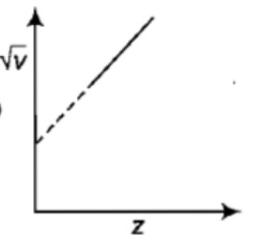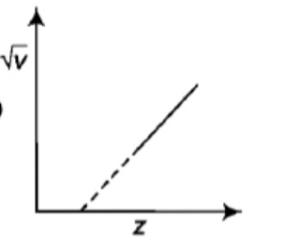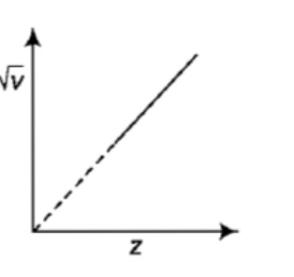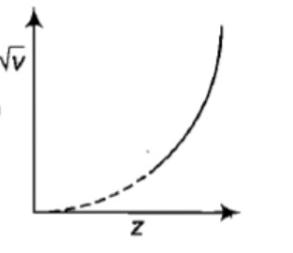You’ve reached the end of your free Videos limit.
#10 | Solved Problems on X Rays
(Physics) > Atoms
Unable to watch the video, please try another server
Related Practice Questions :

An x-ray tube is operating at 30 kV then the minimum wavelength of the x-rays coming out of the tube is:

(1) 1.24 $\stackrel{0}{A}$          (2) 0.413 $\stackrel{0}{A}$             (3) 0.124 $\stackrel{0}{A}$             (4) 0.13 $\stackrel{0}{A}$

High Yielding Test Series + Question Bank - NEET 2020

Difficulty Level:

The minimum wavelength of X-rays produced by electrons accelerated by a potential difference of V volt is equal to

(1) $\frac{eV}{hc}$               (2) $\frac{eh}{cV}$               (3) $\frac{hc}{eV}$               (4) $\frac{cV}{eh}$

High Yielding Test Series + Question Bank - NEET 2020

Difficulty Level:

The graph between the square root of the frequency of a specific line of the characteristic spectrum of X-rays and the atomic number of the target will be:

(1)(2)(3)(4)High Yielding Test Series + Question Bank - NEET 2020

Difficulty Level:

The wavelength of ${K}_{\alpha }$ X-rays for lead isotopes  respectively. Then

(1) ${\lambda }_{1}={\lambda }_{2}>{\lambda }_{3}$                         (2) ${\lambda }_{1}>{\lambda }_{2}>{\lambda }_{3}$

(3) ${\lambda }_{1}<{\lambda }_{2}<{\lambda }_{3}$                         (4) ${\lambda }_{2}={\lambda }_{1}={\lambda }_{3}$

High Yielding Test Series + Question Bank - NEET 2020

Difficulty Level:

which of the following is positively charged?

a.

b.

c.

d.  $\mathrm{X}-\mathrm{rays}$